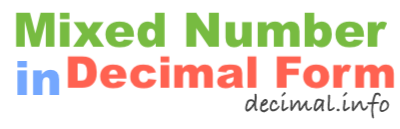What is 1 1/2 in decimal form?To start off, note that 1 1/2 is a mixed number, also known as a mixed fraction. It has a whole number and a fractional number. We have labeled the parts of the mixed number below so it is easier to follow along.

1 = Whole number
1 = Numerator
2 = Denominator

To get 1 1/2 in decimal form, we basically convert the mixed number to a fraction and then we divide the numerator of the fraction by the denominator of the fraction. Here are the detailed math steps we use to convert 1 1/2 mixed number to decimal form:

Step 1: Multiply the whole number by the denominator:

1 × 2 = 2

Step 2: Add the product you got in Step 1 to the numerator:

2 + 1 = 3

Step 3: Divide the sum from Step 2 by the denominator:

3 ÷ 2 = 1.5

That's it folks! The answer to 1 1/2 in decimal form is displayed below:

1 1/2 = 1.50

Mixed Number in Decimal Form
1 1/2 in decimal form is not all we can do! Here you can convert another mixed number to decimal form.

What is 1 1/3 in decimal form?
Here is the next mixed number on our list that we have converted into decimal form.